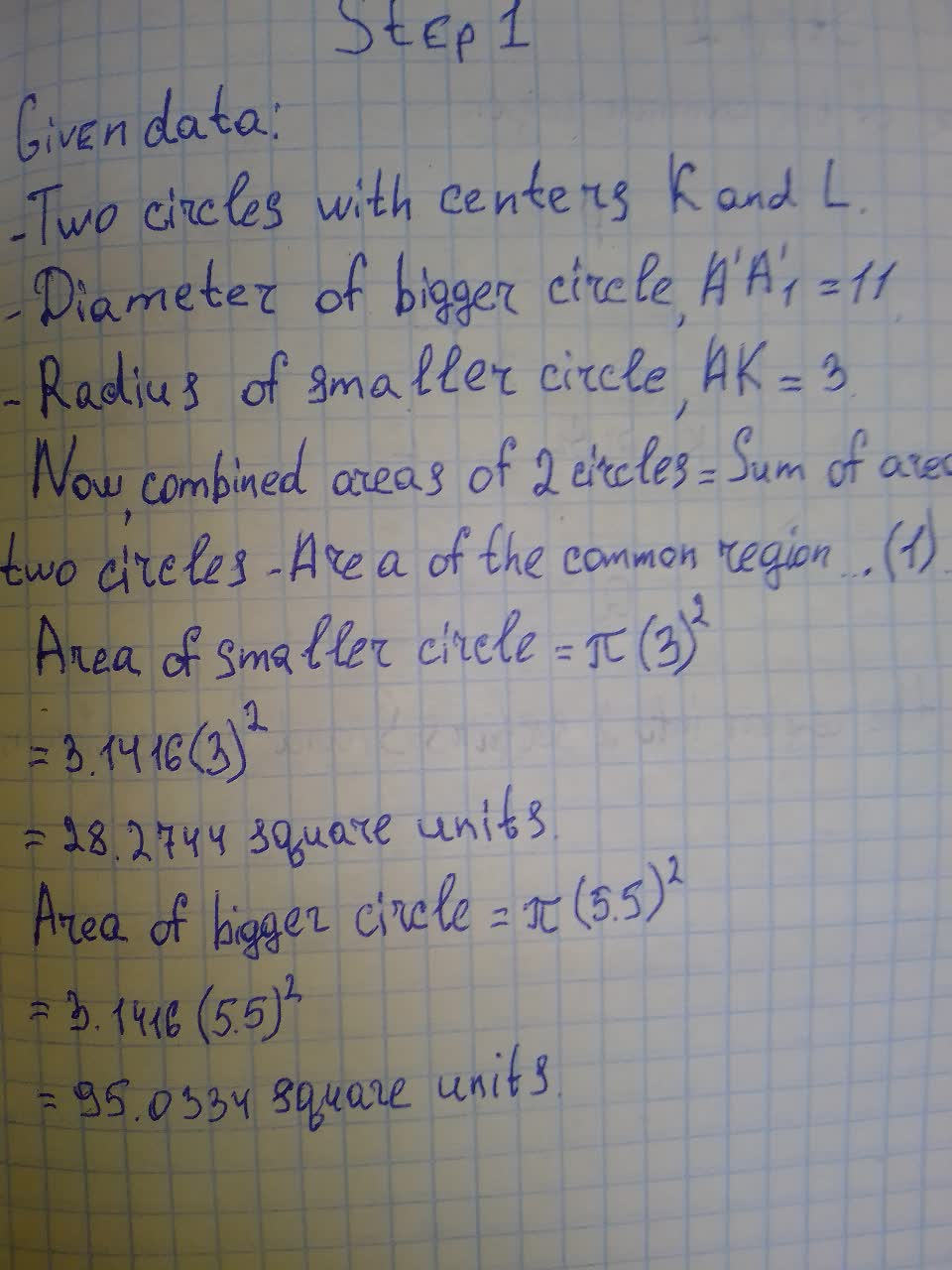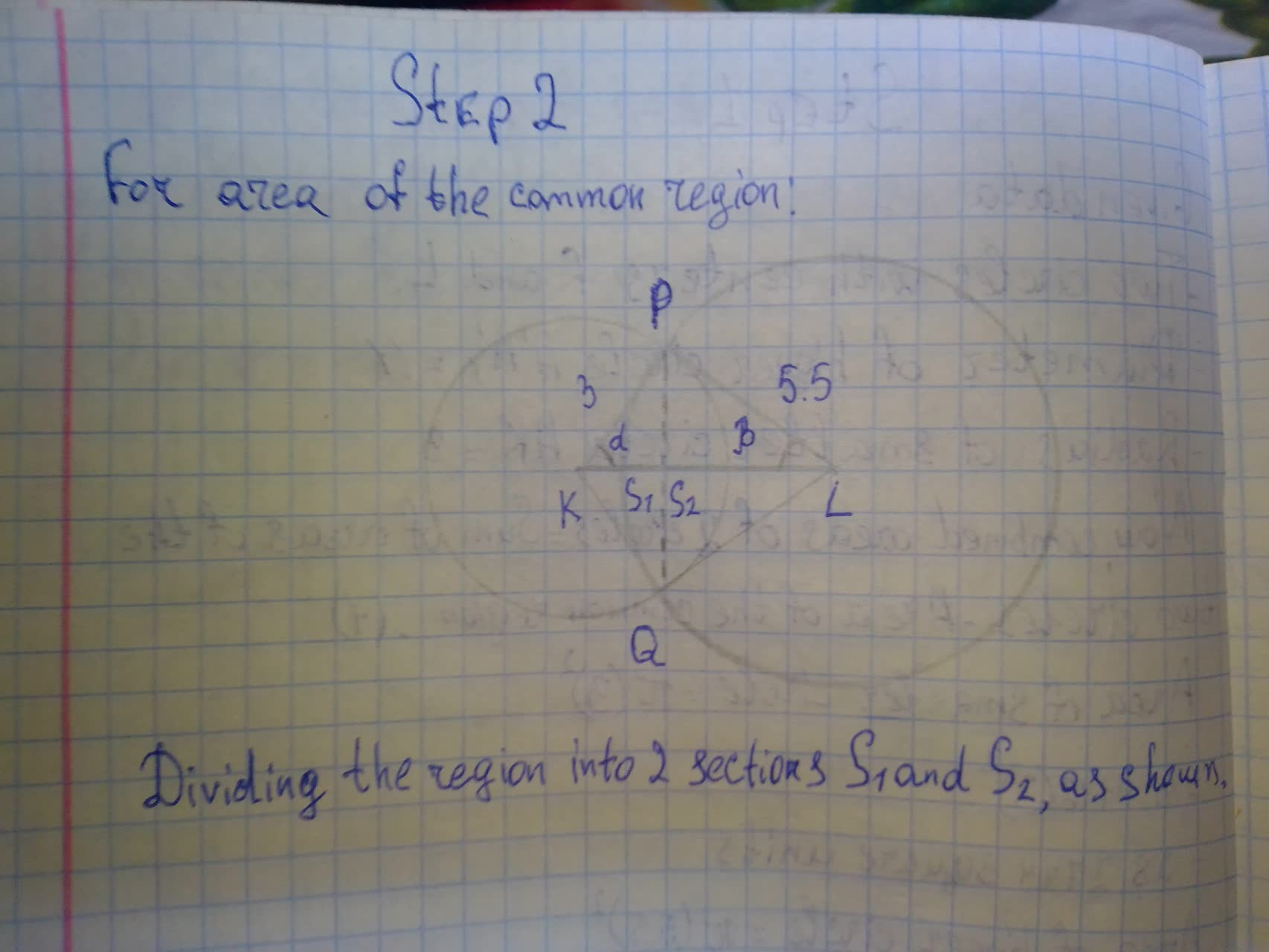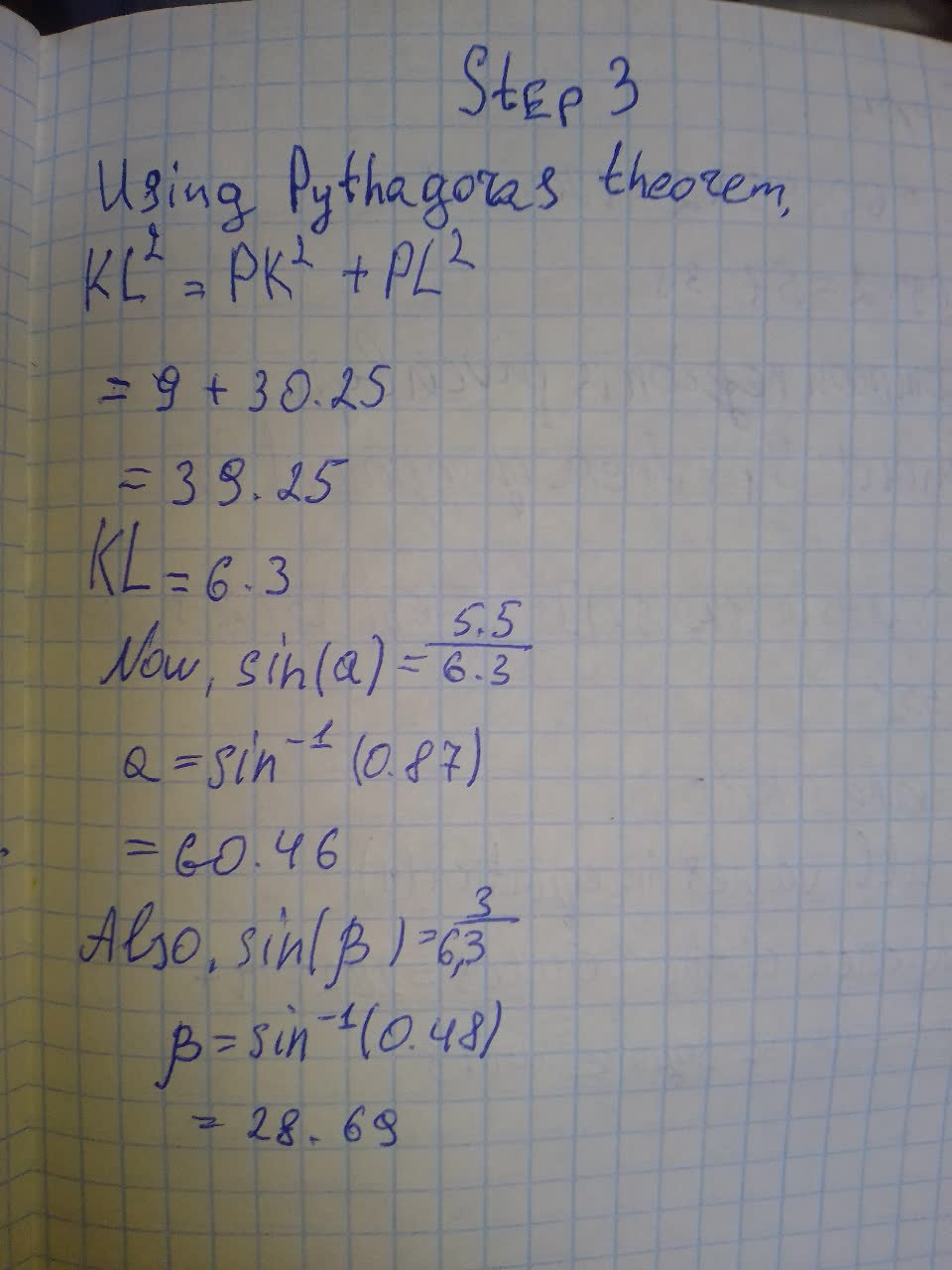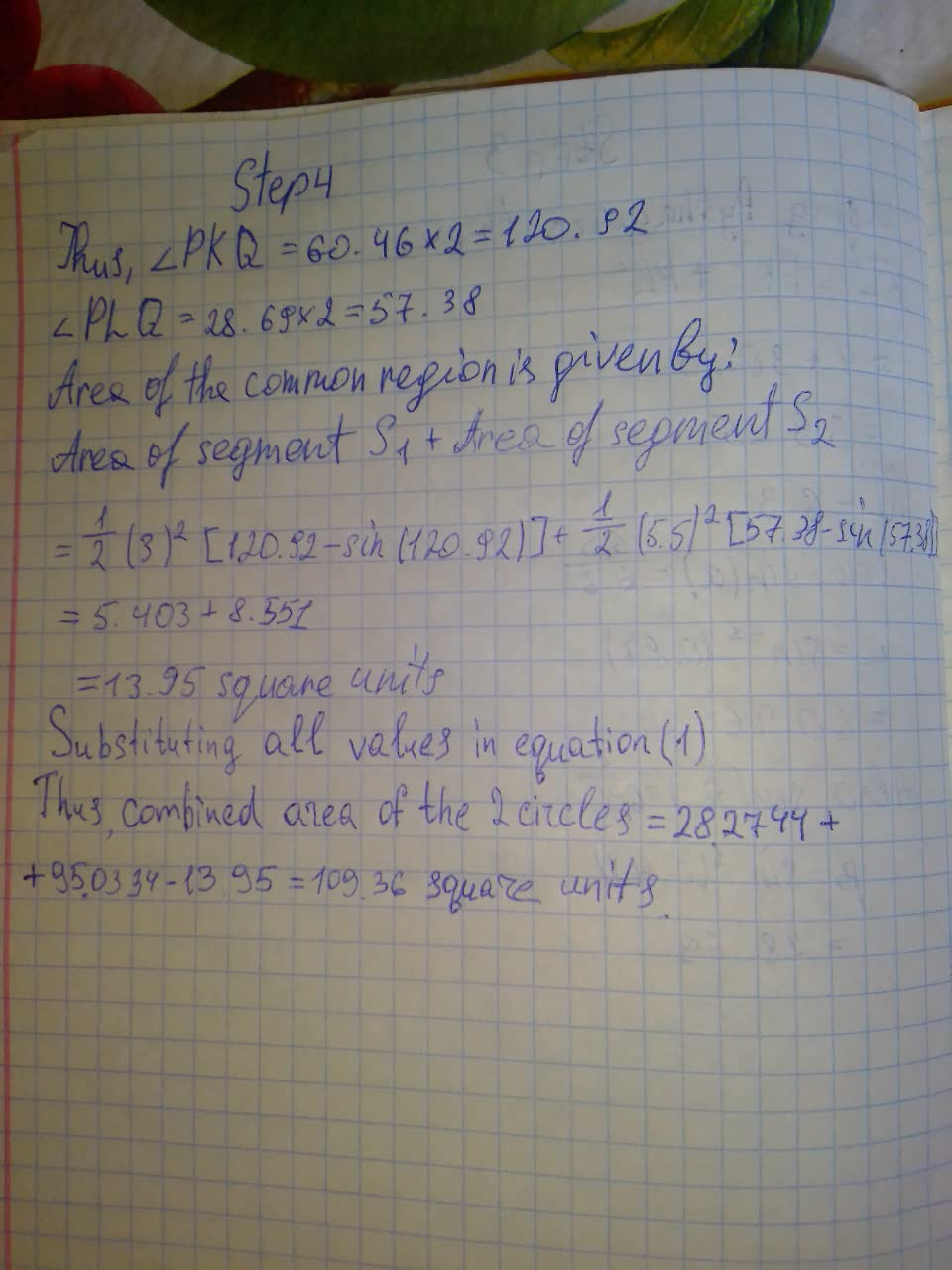Question# Given two circles with centres K and L, AA_1=11 and AK=3, calculate the combained areas of the two circles. Provide answer in whole number.

Circles
ANSWEREDGiven two circles with centres K and L, AA_1=11 and AK=3, calculate the combained areas of the two circles. Provide answer in whole number.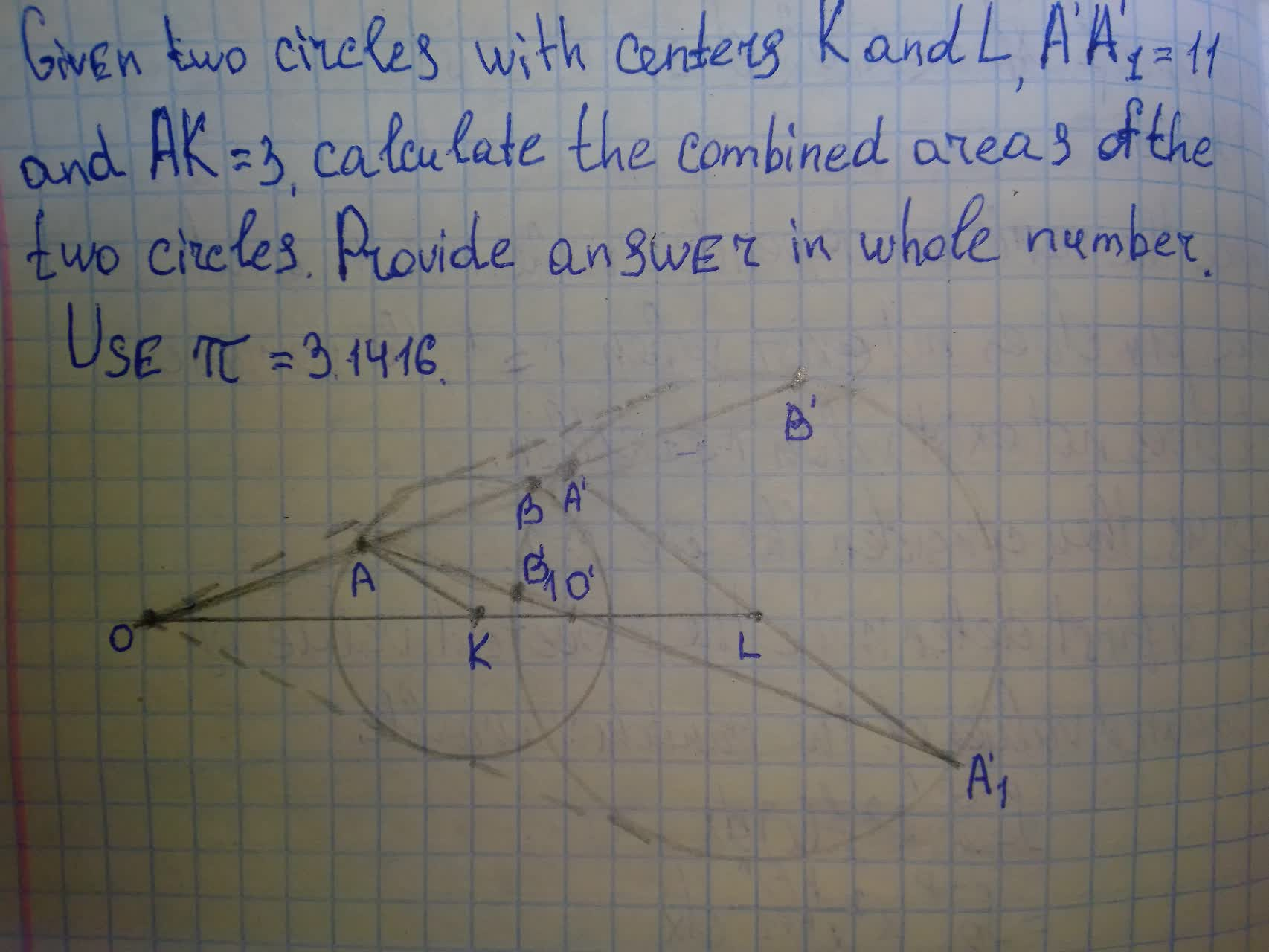2021-08-10